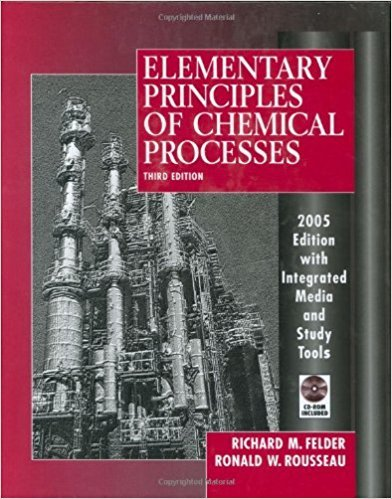×
Get Full Access to Elementary Principles Of Chemical Processes - 3 Edition - Chapter 9 - Problem 9.44
Get Full Access to Elementary Principles Of Chemical Processes - 3 Edition - Chapter 9 - Problem 9.44

×

# A dilute aqueous solution of sulfuric acid at 25C is usedISBN: 9780471687573 143

## Solution for problem 9.44 Chapter 9

Elementary Principles of Chemical Processes | 3rd Edition

• Textbook Solutions
• 2901 Step-by-step solutions solved by professors and subject experts
• Get 24/7 help from StudySoup virtual teaching assistantsElementary Principles of Chemical Processes | 3rd Edition

4 5 1 329 Reviews
29
0
Problem 9.44

A dilute aqueous solution of sulfuric acid at 25C is used to absorb ammonia in a continuous reactor, thereby producing ammonium sulfate, a fertilizer: Student Workbook Encyclopedia Equipment scrubber (a) If the ammonia enters the absorber at 75C. the sulfuric acid enters at 25C, and the product solution emerges at 25C, how much heat must be withdrawn from the unit per mol of (N~)s04 produced? (All needed physical property data may be found in Appendix B.) (b) Estimate the final temperature if the reactor of part (a) is adiabatic and the product of the solution contains 1.00 mole % ammonium sulfate. Take the heat capacity of the solution to be that of pure liquid water [4.184 kJ/(kgC)]. (c) In a real (imperfectly insulated) reactor, would the final solution temperature be less than, equal to, or greater than the value calculated in part (b), or is there no way to tell without more information? Briefly explain your answer.

Step-by-Step Solution:
Step 1 of 3

Chapter 3 ● Energy and Energy Changes ○ Energy is the capacity to do work or transfer heat ○ Forms of Energy ■ Kinetic Energy is energy that results from motion 1 ● E = k mu 2 2 ○ m = mass ○ u = velocity ■ Thermal energy is the energy of random motion of atoms and molecules. ■ Potential energy is the energy of an object based of its position ■ Chemical energy is energy stored within the structural units of chemical substances ■ Electrostatic energy is potential energy that results from the interaction of

Step 2 of 3

Step 3 of 3

##### ISBN: 9780471687573

Since the solution to 9.44 from 9 chapter was answered, more than 438 students have viewed the full step-by-step answer. Elementary Principles of Chemical Processes was written by and is associated to the ISBN: 9780471687573. The full step-by-step solution to problem: 9.44 from chapter: 9 was answered by , our top Chemistry solution expert on 11/15/17, 02:42PM. This full solution covers the following key subjects: solution, reactor, acid, sulfate, ammonia. This expansive textbook survival guide covers 13 chapters, and 710 solutions. The answer to “A dilute aqueous solution of sulfuric acid at 25C is used to absorb ammonia in a continuous reactor, thereby producing ammonium sulfate, a fertilizer: Student Workbook Encyclopedia Equipment scrubber (a) If the ammonia enters the absorber at 75C. the sulfuric acid enters at 25C, and the product solution emerges at 25C, how much heat must be withdrawn from the unit per mol of (N~)s04 produced? (All needed physical property data may be found in Appendix B.) (b) Estimate the final temperature if the reactor of part (a) is adiabatic and the product of the solution contains 1.00 mole % ammonium sulfate. Take the heat capacity of the solution to be that of pure liquid water [4.184 kJ/(kgC)]. (c) In a real (imperfectly insulated) reactor, would the final solution temperature be less than, equal to, or greater than the value calculated in part (b), or is there no way to tell without more information? Briefly explain your answer.” is broken down into a number of easy to follow steps, and 157 words. This textbook survival guide was created for the textbook: Elementary Principles of Chemical Processes, edition: 3.

Unlock Textbook Solution